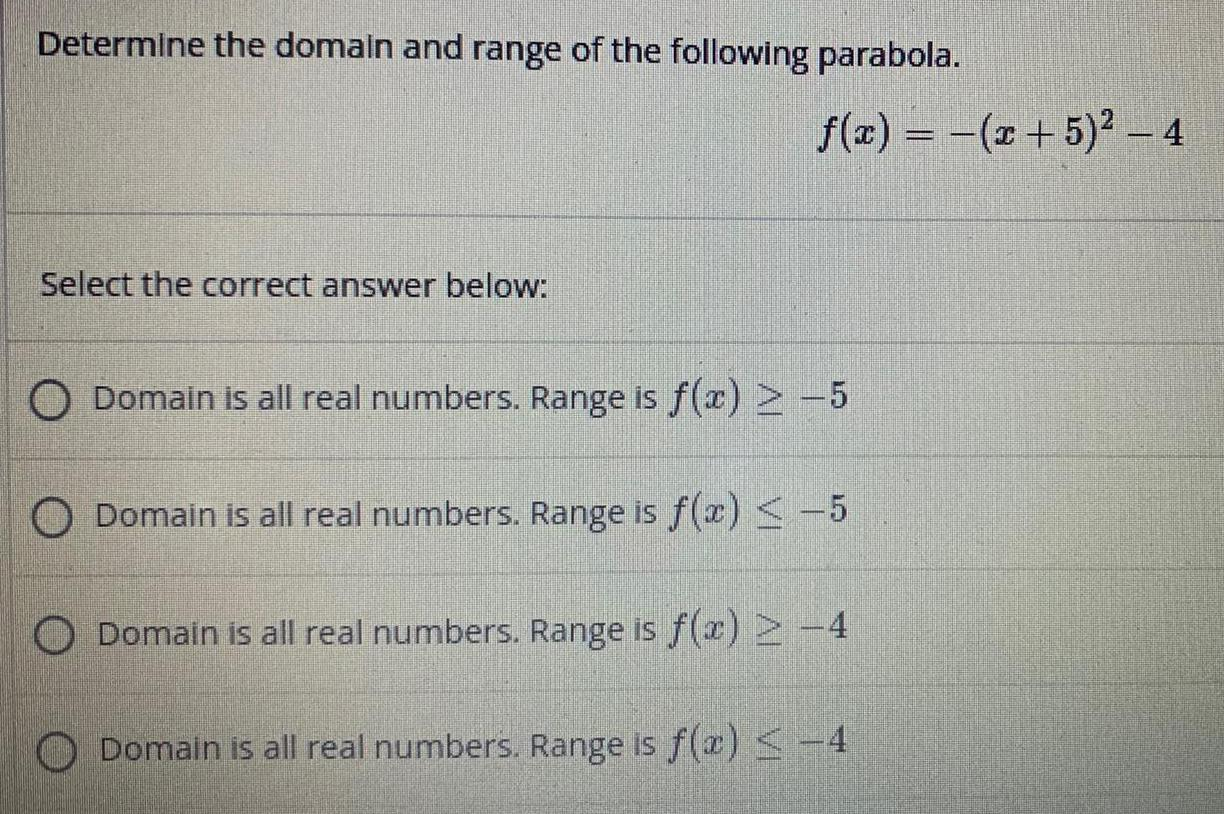Question:

# Determine the domain and range of the following parabola.

Last updated: 7/28/2022Determine the domain and range of the following parabola. Select the correct answer below: f(x) = -(x + 5)² - 4 Domain is all real numbers. Range is f(x) ≥ -5 Domain is all real numbers. Range is f(x) ≤ -5 Domain is all real numbers. Range is f(x) ≥-4 Domain is all real numbers. Range is f(a) ≤-4